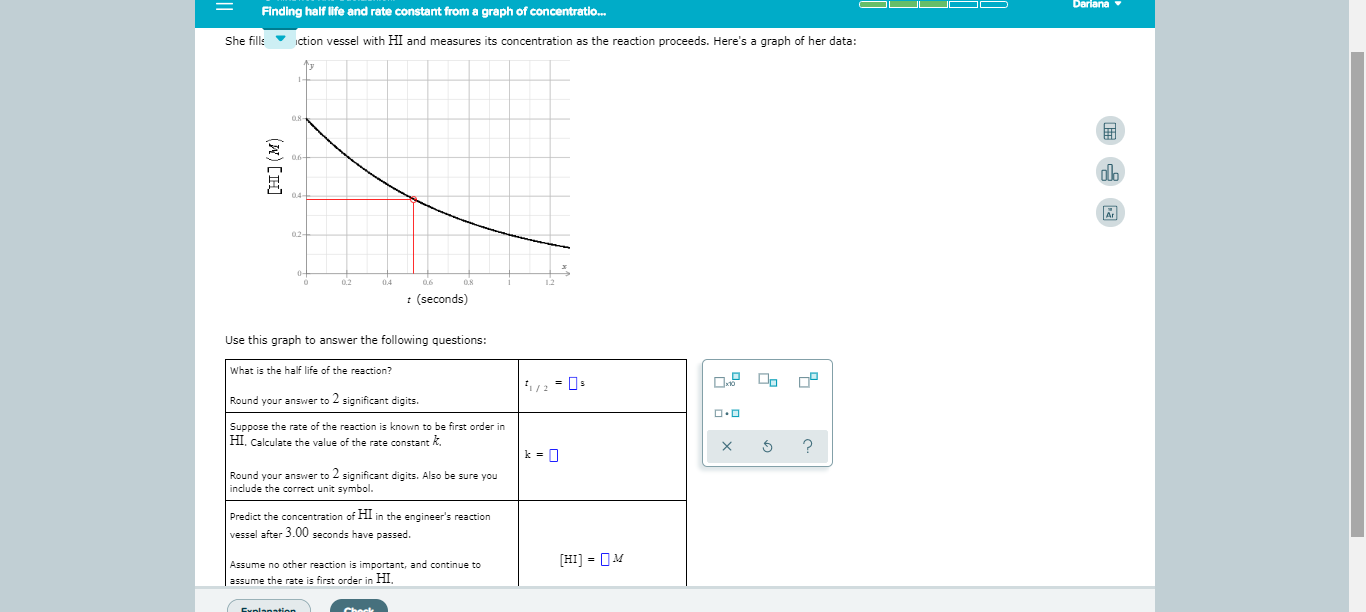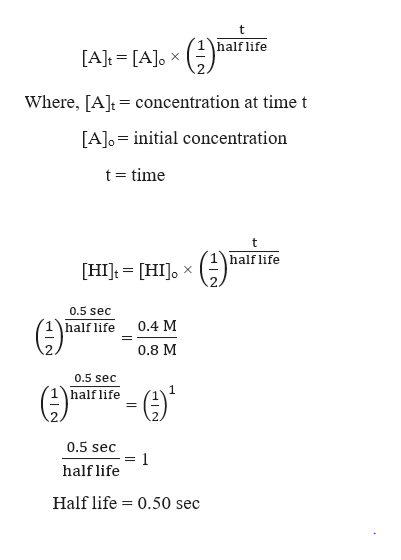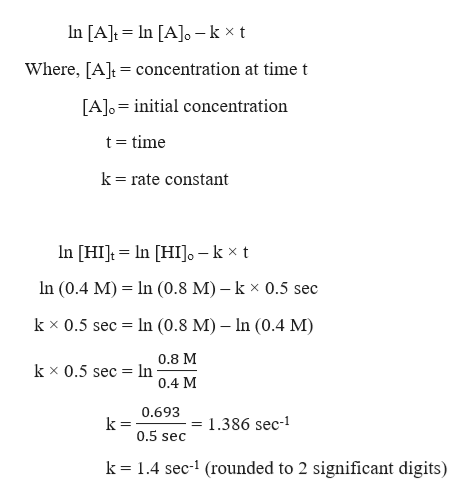# DarianaFinding half life and rate constant from a graph of concentratio...ction vessel with HI and measures its concentration as the reaction proceeds. Here's a graph of her data:She fills(seconds)Use this graph to answer the following questions:What is the ha lf life of the reaction?Round your answer to 2 significant digits.Suppose the rate of the reaction is known to be first order inHI Calculate the value of the rate constant KXk = ]Round your answer to 2 significant digits. Also be sure youinclude the correct unit symbol.Predict the concentration of HI in the engineer's reactionvessel after 3.00 seconds have passed.HI MAssume no other reaction is important, and continue toassume the rate is first order in HI,Expianation(w) H

Question
6 viewshelp_outlineImage TranscriptioncloseDariana Finding half life and rate constant from a graph of concentratio... ction vessel with HI and measures its concentration as the reaction proceeds. Here's a graph of her data: She fills (seconds) Use this graph to answer the following questions: What is the ha lf life of the reaction? Round your answer to 2 significant digits. Suppose the rate of the reaction is known to be first order in HI Calculate the value of the rate constant K X k = ] Round your answer to 2 significant digits. Also be sure you include the correct unit symbol. Predict the concentration of HI in the engineer's reaction vessel after 3.00 seconds have passed. HI M Assume no other reaction is important, and continue to assume the rate is first order in HI, Expianation (w) H fullscreen
check_circle

Step 1

From the given graph, we can predict that,

At t = 0 sec, initial concentration, [HI]o = 0.8 M

At t = 0.5 sec, concentration, [HI]t = 0.4 M

Step 2

Part 1:

Half life of a reaction is the time at which the initial concentration of the reactant becomes half. So, half life will be 0.5 sec as the initial concentration (0.8 M) becomes half (0.4 M)

Also, the half-life of the reaction can be calculated as using the following formula:help_outlineImage Transcriptioncloset half life [A] [A]o Where, [At concentration at time t [A]o initial concentration t time half life [HI [HI] X 0.5 sec 0.4 M half life 0.8 M 0.5 sec -G) 1 half life 0.5 sec 1 half life Half life 0.50 sec fullscreen
Step 3

Part 2:

Given, the order of the reaction is 1.

Therefore, the rate constant of the reactio...help_outlineImage TranscriptioncloseIn [AJo k xt In [A]t Where, [Atconcentration at time t [A]o initial concentration t time k rate constant n [HIt In HI]o - k x t In (0.4 M) In (0.8 M) - k x 0.5 sec k x 0.5 sec In (0.8 M) In (0.4 M) 0.8 M In 0.4 M k x 0.5 sec = 0.693 1.386 sec- k= 0.5 sec k 1.4 sec-1 (rounded to 2 significant digits) fullscreen

### Want to see the full answer?

See Solution

#### Want to see this answer and more?

Solutions are written by subject experts who are available 24/7. Questions are typically answered within 1 hour.*

See Solution
*Response times may vary by subject and question.
Tagged in

### Chemical Kinetics